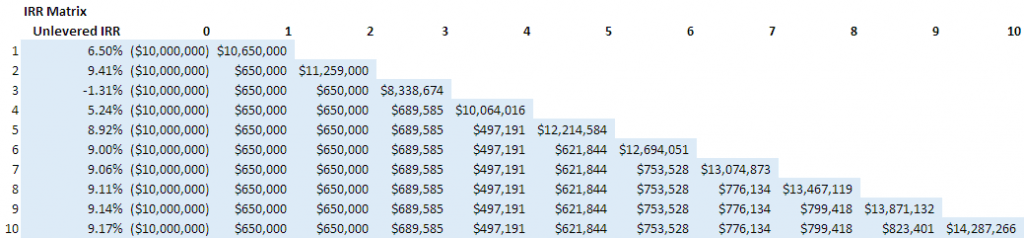# Using an IRR Matrix to Determine Hold Period

When building a real estate financial pro forma, it’s important to model for and include a summary of various return and risk metrics. Among the different return metrics, the internal rate of return (IRR) – both on an unlevered and levered basis – is one of the more important metrics to include in your analysis.

One issue I find in models, however, is that often the internal rate of return will only be calculated for the entire hold period. The problem with doing this, is you’re not necessarily sure that the hold period you’ve selected (e.g. 10 years) maximizes the internal rate of return. One solution to this problem, is to include an IRR matrix into your model that calculates and reports the internal rate of return at the end of each year of the hold period. This way, you can see the projected internal rate of return in any given year helping you to better determine an appropriate hold period.

In this post, I’ll show you how I build an IRR matrix and share an Excel Workbook with an IRR matrix included for you to use in your own real estate financial models.#### What You’ll Need to Build the IRR Matrix

In this example, I’ll build both an investment-level unlevered IRR Matrix (IRR before debt without taking into account partnership split) and an investment-level levered IRR Matrix (IRR after debt without taking into account partnership split). To do this, you’ll first need to model certain cash flows:

1. Model cash outflows (e.g. acquisition costs in time zero)
2. Model NOI (net operating income) one year past analysis period (e.g. if 10 year analysis, than model to year 11)
3. Model debt including initial debt funding, debt service payments and loan payoff at the end of each period
4. Model CFAF (cash flow after financing) through the entire analysis period
5. Set an annual cap rate and model an investment value at the end of each period/year

Note: For simplicity, in this example I use annual periods and model out to year 10.

#### What Next?

Rather than type out the instructions, allow me to show you with a video how I take the above referenced cash flows and quickly build the Matrix using one relatively simple formula. As you’ll see, the key to building the module is using the appropriate absolute and relative cell references in the formula so that you can write just one formula and then copy over and down all at once. Below the video, find a link to the workbook I use in the video to see the formulas first hand. If you have any questions, feel free to reach out.

#### Video Tutorial

Real Estate IRR Matrix Example
• Workbook used in my IRR Matrix tutorial video
• 10 year analysis
• Annual periods
• Unlevered and Levered IRR calculation

Tags: